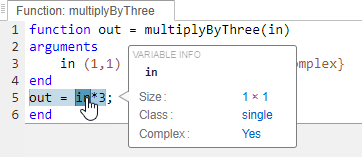# coder.mustBeComplex

Validate that value lies on the complex plane

Since R2023b

## Syntax

``coder.mustBeComplex(value)``

## Description

example

````coder.mustBeComplex(value)` validates that the function input `value` can have a nonzero imaginary part. In MATLAB® execution, this function does not throw any assertion because any numeric input can have a nonzero imaginary part. In code generation, this validator specifies at compile time that `value` has a complex type.```

## Examples

collapse all

This example shows how to specify that an entry-point input has complex type for code generation using the `coder.mustBeComplex` validator.

Define entry-point function `multiplyByThree` that accepts a complex single-precision scalar input.

```function out = multiplyByThree(in) arguments in (1,1) single {coder.mustBeComplex} end out = in*3; end```

Generate a static library for `multiplyByThree` using the `codegen` command. Because the `arguments` block fully specifies the type, size, and complexity of the input argument `in`, you do not need to use the `-args` option.

`codegen -config:lib -c multiplyByThree -report`
`Code generation successful: View report`

Open the code generation report. Observe that the input argument `in` has a complex type.This example shows how to validate that an input to a non-entry-point function has a complex type.

Define the entry-point function `testComplex` that calls the local function `local`. The function `local` accepts one input that is declared to be complex using the `coder.mustBeComplex` validator.

```function y = testComplex(x) y = local(x); end function v = local(u) arguments u {coder.mustBeComplex} end v = u + sqrt(u); end```

Attempt to generate code for the `testComplex` function. Specify the input to be a scalar double using the `-args` option. Code generation fails because the code generator treats the input to `testComplex` as real by default. You pass this real type directly to `local`, thereby triggering an error from the `coder.mustBeComplex` validator.

`codegen testComplex -args 0`
```Value must be complex. Error in ==> testComplex Line: 7 Column: 8 Code generation failed: View Error Report```

To fix this issue, convert the input `x` to `testComplex` to a complex type before passing it to `local` using the `complex` function.

```function y = testComplex(x) y = local(complex(x)); end function v = local(u) arguments u {coder.mustBeComplex} end v = u + sqrt(u); end```

Run the same `codegen` command again.

`codegen testComplex -args 0`
`Code generation successful.`

## Input Arguments

collapse all

Value to validate, specified as a numeric scalar or array.

Data Types: `single` | `double` | `int8` | `int16` | `int32` | `int64` | `uint8` | `uint16` | `uint32` | `uint64`

## Version History

Introduced in R2023b